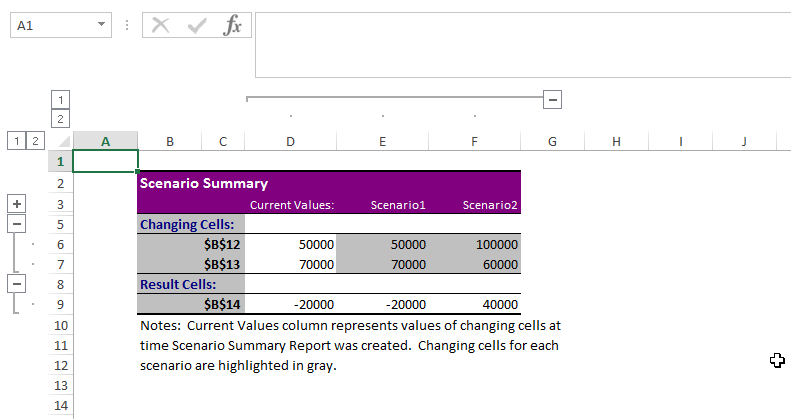## Scenario manager in excel in Excel

Scenario Manager comes under "What If" Analysis of Excel.

As the name indicates, it is a prediction tool that allows users to check output for various expected input values.

Scenario Manager is one among the "What If" Analysis tool that predicts the possible outcome of various scenarios.

A�Scenario�is a set of values that Excel saves and can substitute automatically on worksheet.

If there are several scenarios for which the outcome has to be predicted in order to choose a right plan, then the "Scenario Manager" can be of very much use.

Collect all possible values and substitute the values to see the possible output.

The following example illustrates this:

Financial Prediction 2017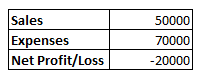From the above example, let us take two cases of Sales and Expense to predict the output Profit/Loss.

Goto "What If" and Select Scenario Manager as shown below: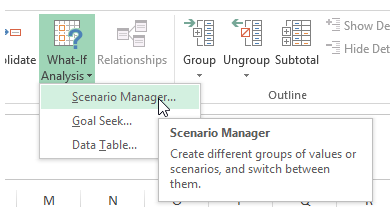The following window appears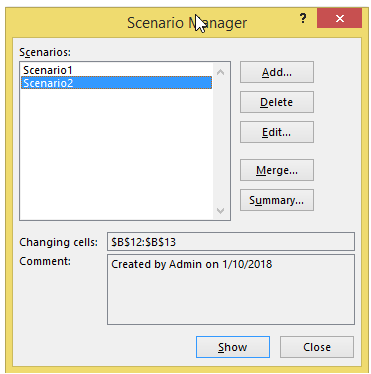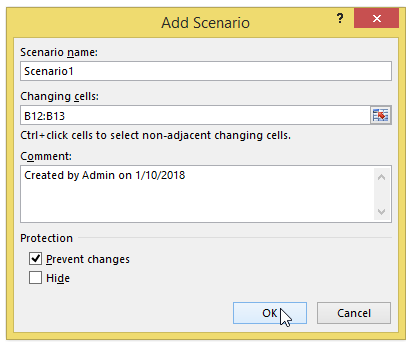Now, give the possible values of Sales and Expense as shown below: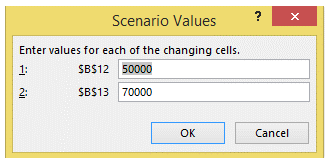Now Click on the "Show" button. It will automatically calculates the Profit/Loss in the Cell B14.

Similarly, a number of Scenarios can be created with various Sales and Expenses.

In this example, we have created two scenarios; Scenario1 and Scenario2.

By clicking on the "Summary" button, a new sheet with the Summary of the results of both the scenarios will be shown as below: